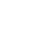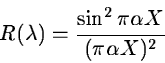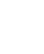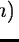Next: Order Merging Up: Instrument Response Correction Previous: Using a Standard Star

Fitting the Blaze Function

A different approach is also available at this stage, as an alternative to the method described in the last Section. It consists of a correction for the blaze function by using a suitable model of the blaze effect introduced by the echelle grating. In this approach, no correction for the chromatic response of the instrument is applied. It is noteworthy however to say that the standard star correction is a much more efficient way to perform the instrumental response correction. The model assumes a plane grating, used in near-Littrow mode. The blaze function R at wavelengthis approximated by(7.5)

whereis a grating constant' with value between 0.5 and 1, and, in which m is the order number, andis the central wavelength of order m. Both parameters are related through the grating constant' k by. This correction is done with the command RIPPLE/ECHELLE; the command includes three methods to compute the parameters k and:

The first one, method SINC, is a modification of the method suggested by Ahmad, 1981, NASA IUE Newsletter, 14, 129. This algorithm approximates the blaze function by a sinc square and finds the function parameters by a non-linear least squares fit to the order profile. The method is suitable for objects without strong emission or absorption features and can be used to get a first estimation of the blaze parameters.

The second method, named OVER, is based on Barker, 1984, Astron.J., 89, 899. This method uses the overlapping region of adjacent orders to estimate, in a few iterations, the parameter k of the blaze function which is, as before, assumed to be a sinc square. The method works well, provided that orders are overlapping and that there is a very good estimation of the parameter, assumed to be a contant.

The third method, FIT, is an extension of the previous one. It uses, as before, the overlapping region of the adjacent orders but has the advantage of assuming that both parameters k andcan vary. The method minimises the difference of the corrected orders in each of the overlapping intervals.Next: Order Merging Up: Instrument Response Correction Previous: Using a Standard Star
Petra Nass
1999-06-15